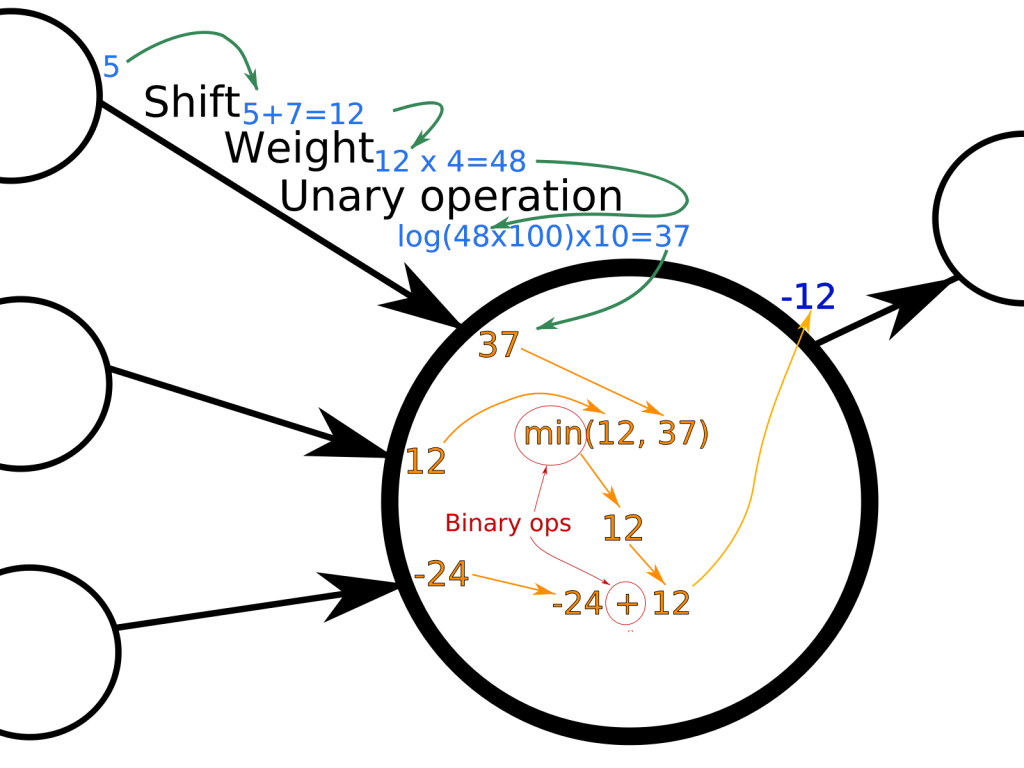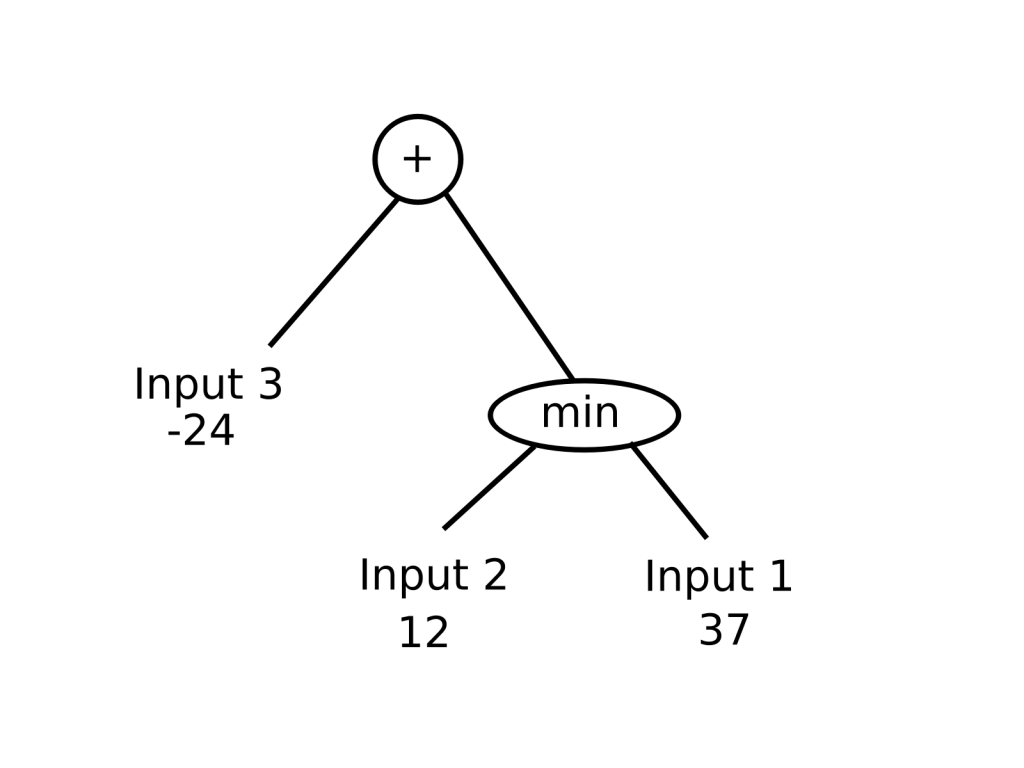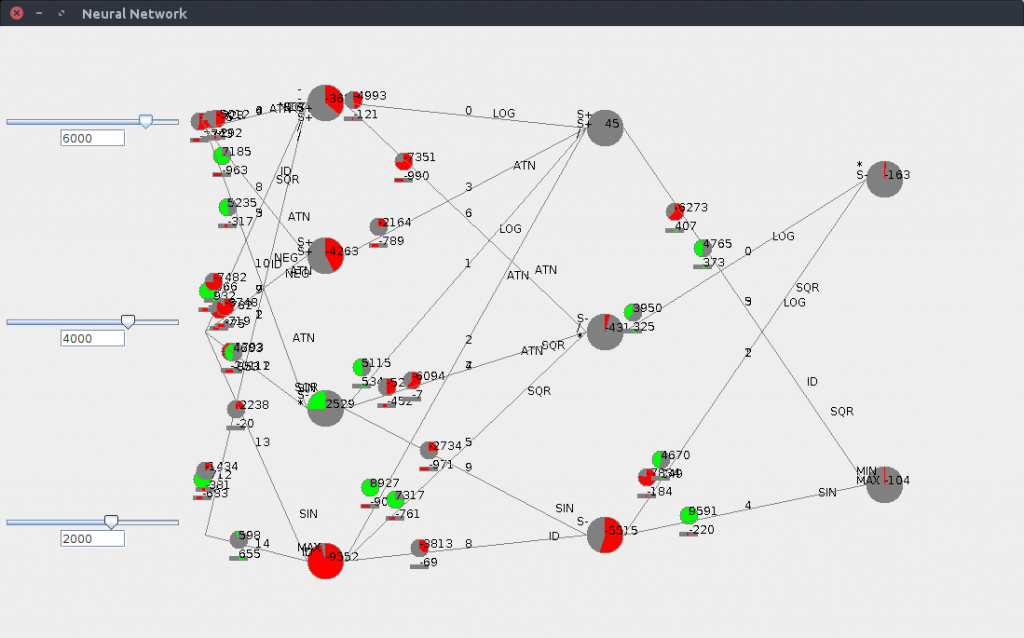# GANN-EWN / Part 3 / building a Neural Network from scratch

Welcome to the third part of the GANN-EWN (Genetic Algorithms for Neural Networks applied to the game “Einstein Würfelt Nicht”) series! If you haven’t read it yet, it’s probably time to read the first part and/or the second part.

In this part, we’ll start building a Neural Network from scratch.

I assume that you’re familiar with Neural Networks, which are basically a simplified stereotype of what people thought neurons in the brain were like in the 1950’s. 🙂 Since then, biological research has evolved a lot and we know now that real neurons and their interactions are much more complex than the general models of Computer Neural Networks that are around.

Anyway, there are many types of neural networks around, all used for different tasks. My attempt is to build a very generic neural network structure that can then be used in various environments, typically to play different games.

# Important structural decisions

Since the early days of neural network research, the transfer functions used in artificial neurons have been carefully chosen to enable backpropagation. In here, though, we want to use our neural network through Genetic Algorithms by selecting the best neural networks within a population, and we won’t necessarily be using backpropagation to make the network “learn”. So we can use any kinds of transfer functions we want.

At first, I also wondered if I would be using totally random graphs for my network structure, but it poses problems such as cycles which require more computational power to deal with so I stayed with a “simple” layer architecture. But because I wanted to code a network that would be as generic as possible, the only constraints I kept was to have a structure with layers: links between neurons of different layers can be as random as possible, with the only requirement that every neuron should have at least one input and one output (a neuron without any input or output would simply be useless and would waste resources unnecessarily). On the other hand, I didn’t put any restrictions on duplicate links from one neuron to another. After all, it could be useful to have the sinus of something and combine it with its raw value.

Another important decision was to limit to the strict minimum the manipulation of floating point values. There are two reasons for this, which are both linked to the projected use of GPUs for my research, and based on my own experience of using GPUs. The first one is that GPUs in general are not so great, performance-wise, with floating point values. The second is that the results of floating point operations may differ between CPU operations and GPU operations, due to rounding and approximating some functions on the GPU. Besides, most GPUs have limited support for double floating point operations, which makes portability some kind of a pain. We will actually discuss that in more detail in another article.

So I decided to take only integers as neuron and link input and output values, as well as the weights and shifts that could be applied on all operations. However, some operations will need some floating point numbers, such as sigmoid and tangent functions, but the results will be transformed back into integers as soon as possible so that floats are used as little as possible.

# Description of neurons and links

Besides, as I didn’t stick with “simple” linear or sigmoid functions for my neurons, the links themselves also have interesting properties. Here is a simple figure showing the basics of my neurons and links:So the output of every link is of the form: out = unary_op ( (in + shift) x weight )

As for computing the result of a neuron, I used what is generally referred to as “genetic programming” where the genetic algorithm doesn’t mutates “parameters” for a program, but rather a tree of instructions (a program). So in my case, a neuron contains a tree of mathematical operations to be applied on the inputs.

So the output of a neuron having n inputs is:

• the input itself if n==1
• the result of a tree of n-1 binary operations

For instance, the tree for the previous picture is the following:The unary operations I have chosen are: id (identity), sinus, log, square, square root, tan, and negative.

The roughly described binary operations are:

• add (actually average, we’ll see why later),
• divide (leave numerator unchanged if denominator is 0),
• subtract,
• multiply (with an adjusting weight),
• max and min,
• an hyperbolic paraboloid and its reverse.

With this in mind, it is actually not difficult to switch this network to any type of network, including simple linear functions. Just limit the allowed operations to “+” and we’re pretty good to go. The same goes with links, just restrict them to use “identity” as their unary operations and set the weights as desired, just leave the offset (often called “bias” in neural network computing).

Besides, as the link structure is not constrained either, we can create any type of network we want by forcing the links to be in a certain way when we build the network.

# Integers and range

There is one remaining problem here: if you store everything as integers and never stop adding and multiplying numbers, you’ll overflow at some point.

So I kept all operations to be within a certain range and added weights and min/max to make sure that all numbers would be kept within that range. How to define the range? It has to be as big as possible to allow for enough precision (obviously if we keep only integer values from -10 to 10, we won’t be able to store Pi using a good precision, but if we go up to a million, then we can store 3.14159, which is already not that bad). On the other hand, the range should allow not to lose precision while doing operations such as multiplying integers. Integers in Java or OpenCl have a rough range of -2 billion to 2 billion so 10.000 should do it (the square is 100 million, perfectly in range).

So within the network, all values circulating should be within the -10.000 to 10.000 range, which means that some adjustments had to be made on the different binary and unary operations, in order to always keep results within that range. For instance, adding is converted to an average, to make sure the result is still within the desired range.

# Encoding and storing the networks

Because the ultimate goal is to run this on GPUs, the networks cannot be programmed as Objects. Instead, plain arrays of integers are used. One other advantage is that it is very simple to store an array of integers and it could be manipulated in any programming language (provided that we code the program that would interpret this array).

Here is the description of the array describing a network so far:

• : the number of layers L, including the output which is considered as a layer, but excluding the input,
• [1 : L]: the number of neurons in each layer (including the output layer, but excluding the input),
• [L+1 : 2xL]: the number of links between each layer (at position L+1, we have the number of links between the input and the first hidden layer),
• [2xL+1:…]: all link parameters (we will go over them shortly),
• after the links: all binary operations for the links, for every layer connectivity there are as many as links minus one.

Every link contains 5 integers and is described as follows:

• the number of the source neuron in the source layer (this number is unique within the network, so the number of the first neuron of the first hidden layer is equal to the number of inputs),
• the number of the destination neuron in the destination layer,
• the applied offset on incoming values,
• the applied weight applied on the result of the offset,
• the code for the unary operation applied to that result.

Let’s calculate the size of an array needed to encode the following network: 20 connections between inputs and the first hidden layer, 10 neurons in the first hidden layer, 30 connections from the first hidden layer to the second hidden layer, 4 neurons in the second hidden layer, 10 connections from the second hidden layer to the 2 outputs.

1 + 2 x 2 + 5 x 50 + 19 + 29 = 303 elements

For now, the networks are stored within a simple local H2 database.

# Watching it in action

Finally, I built a small UI to watch how the network behaves when its inputs change. Later, it might come in handy to see how the network behaves during a real game:The inputs are on the left, and can be entered either numerically or from sliders, and the outputs are read on the right (this one has only two outputs). The two hidden layers (containing 4 and 3 neurons) are clearly visible. Besides, every link has the offset represented as a bar (red means negative, green means positive, full gray is 0) and the weights are represented as filled circles.

In the next article, we’ll build the genetic algorithm part of the project.

The next post of the series is here.